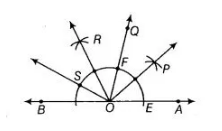# Draw an angle of 80° with the help of protractor.`
Question:

Draw an angle of 80° with the help of protractor. Then, construct angles of (1) 40° (2) 160° and (3) 120°.

Solution:

First, draw an angle of 80° say ∠QOA = 180° with the help of protractor. Now, use the the following steps to construct angles of(1)40° 2() 160° (3) 120°

1. Taking O as centre and any radius draw an arc which intersect OA at E and OO at F.
2. Taking E and F as centres and radius more than ½ EF draw arcs which intersect each other at P.
3. Join OP Thus, ∠POA = 40° [∴ 40° = ½ x 80°]
4. Now, taking F as centre and radius equal to EF draw an arc which intersect previous arc obtained in step ii at S.
5. Join OS. Thus, ∠SOA = 160° [∴ 160° = 2 x 80°]
6. Taking S and F as centre and radius more than ½ SF draw arcs which intersect each other at R.
7. Join OR. Thus, ∠ROA = ∠ROQ = 40° + 80°= 120°.